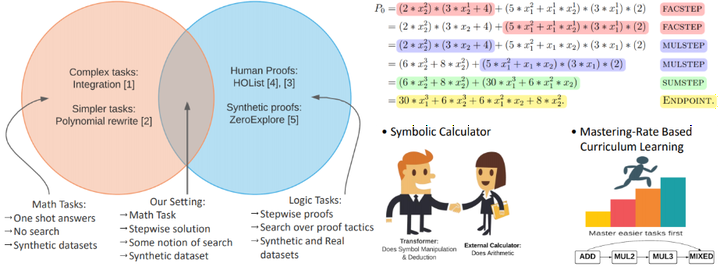# Analyzing the Nuances of Transformers' Polynomial Simplification AbilitiesType
Publication
In ICLR 2021 MathAI Workshop

Symbolic Mathematical tasks such as integration often require multiple welldefined steps and understanding of sub-tasks to reach a solution. To understand Transformers’ abilities in such tasks in a fine-grained manner, we deviate from traditional end-to-end settings, and explore a step-wise polynomial simplification task. Polynomials can be written in a simple normal form as a sum of monomials which are ordered in a lexicographic order. For a polynomial which is not necessarily in this normal form, a sequence of simplification steps is applied to reach the fully simplified (i.e., in the normal form) polynomial. We propose a synthetic Polynomial dataset generation algorithm that generates polynomials with unique proof steps. Through varying coefficient configurations, input representation, proof granularity, and extensive hyper-parameter tuning, we observe that Transformers consistently struggle with numeric multiplication. We explore two ways to mitigate this: Curriculum Learning and a Symbolic Calculator approach (where the numeric operations are offloaded to a calculator). Both approaches provide significant gains over the vanilla Transformers-based baseline.